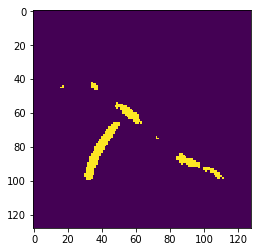# Batch prediction on inference learner for image segmentation

I trained a learner to predict the masks of some images, and now I would like to deploy it. I am currently predicting one image at a time using

``````learn = load_learner(path_learner, 'export.pkl')

for i in range(curr):
img = open_image(f'{test_dir}/{i}.png')
img.resize(128)
``````

But it becomes quite slow when the number of images increases, so I would ideally like to do batch predictions via

``````learn = load_learner(path_learner, 'export.pkl', test = SegmentationItemList.from_folder(\$TEST_DIR))
preds = learn.get_preds(ds_type=DatasetType.Test)
``````

However I am getting

``````Exception: Attempting to apply transforms to an empty label. This usually means you are
trying to apply transforms on your xs and ys on an inference problem, which will give you wrong
predictions. Pass `tfms=None, tfm_y=False` when creating your test set.
``````

I’ve looked into this thread for solutions, but it sounds like the only solution is to create a new databunch

``````src = (SegmentationItemList.from_folder(path_img)
.split_by_rand_pct(0.2)
.label_from_func(get_y_fn, classes=codes))

data = (src.transform(get_transforms(flip_vert = True, max_rotate=180), size=size, tfm_y=True)
.databunch(bs=bs)
.normalize(imagenet_stats))

learn = unet_learner(data, models.resnet50, metrics=metrics, wd=wd)

``````

But the problem is if I’m deploying this on another computer, I would have to transfer all the data folders + the `weights.pth` instead of just an `export.pkl`

Is there any workaround to this issue?

1 Like

You can pass `tfm_y=False` to `load_learner` to avoid the error, but I don’t know what your transforms are so the predictions (which will be done on transformed images) might not correspond to your inputs. You should export your `Learner` after changing its `DataBunch` with a version that doesn’t have transforms to be safe (note that this requires having all your images at inference having the same size).

Does `get_preds` keep the order of the test data? Because I ran

``````learn = load_learner(path_learner, 'export.pkl', test=SegmentationItemList.from_folder(save_dir), tfm_y = False)

preds, y = learn.get_preds(ds_type=DatasetType.Test)

``````

And the first image `mask` isBut if I run

``````for i in range(curr):
img = open_image(f'{save_dir}/{i}.png')
img.resize(128)
``````

The first image (mask_0) comes out to beMaybe it has something to do with the transformations? Since I am scaling the input images to be 128x128

Edit: upon further digging, it looks like the data is being shuffled when passing in the test dataset. My data is ordered such that 0.png is followed by 1.png etc. Is there a way to keep the order when doing batch predictions?

1 Like

That is weird, normally the test dataloader is not shuffled. Are you sure your test items (dbunch.test_ds.x.items) are in the order you say?

You’re right, there’s some issue between the order they appear in the directory and what’s in `os.listdir`

One other question: if I run

``````mask_pred = learn.predict(img)
``````

the `type(mask_pred)` is a `class 'fastai.vision.image.ImageSegment'`

But when I do

``````preds, y = learn.get_preds(ds_type=DatasetType.Test)
``````

`type(mask)` is instead a `torch.Tensor`. Can I convert a torch tensor into an Image class so that I can use `mask.save('mask.png')`

Can’t help with the first one. But on the second turn it into a numpy array and then you can create a PILImage out of it. IE: `im = mask.cpu().numpy()` and then

``````plt.imshow(im)
``````

Let me know if this doesn’t and what error it gives

There appears to be a mismatch between the 2 methods

If I use `mask.save('mask.png')` the image comes out toWith `matplotlib.image.imsave('mask.png', mask)` The image comes out toWhich messes up some steps further down the pipeline. I would like to have the mask image in the same format as the 1st image, particularly for using the fastai `mask.resize()` method

Facing the same issue. Predictions are correct but in random order.
Tries ordered = True but it woks only for the text models.
I found several other threads with the same issue.